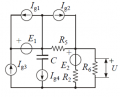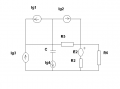# Finding complex voltage in AC circuit.

#### cdummie

Joined Feb 6, 2015
124
I have a circuit shown in the picture below with the following known values:
Ig1=j2mA
Ig3=jmA
Ig4=0.5mA
E2=1V
R2=2kΩ
R5=1kΩ
R6=4kΩ
C=2μF
ω=10^4 s^-1

and i have to find complex value of voltage U shown in the pictureWhen doing examples like this, the easiest way for me is to use Thevenin's theorem, and it could work just fine if i had to find Thevenin impedance, because, when finding Thevenin impedance, every voltage generator is short circuit and every branch with current generator can be removed, so i have that Thevenin impedance is R2, but i don't know how to find Thevenin generator since i have a few unknown values in the circuit.

#### WBahn

Joined Mar 31, 2012
28,184
Look to see if any of the sources are masked, at least as far as R6 is concerned. Naturally, focus on the ones that you don't have values for first.

#### cdummie

Joined Feb 6, 2015
124
Look to see if any of the sources are masked, at least as far as R6 is concerned. Naturally, focus on the ones that you don't have values for first.

I am sorry, but, what do you mean by masked?

#### WBahn

Joined Mar 31, 2012
28,184
If you have a voltage source in series with a current source, the voltage source is masked in that it has absolutely no affect on the circuit voltages and currents (other than the voltage across the current course). Similarly, a current source in parallel with a voltage source is masked.

#### cdummie

Joined Feb 6, 2015
124
If you have a voltage source in series with a current source, the voltage source is masked in that it has absolutely no affect on the circuit voltages and currents (other than the voltage across the current course). Similarly, a current source in parallel with a voltage source is masked.

Ok, thanks, so this means that current through the E1 is Ig1. Does it means that E1 and Ig3 are in series, i mean if Ig1 doesn't affect the rest of the circuit and if that's so, how come that there is current different than Ig3 through E1 (because the current through it is Ig1) even though it is in series with Ig3? If this isn't the case, then what am i doing wrong?

#### WBahn

Joined Mar 31, 2012
28,184
Ok, thanks, so this means that current through the E1 is Ig1. Does it means that E1 and Ig3 are in series, i mean if Ig1 doesn't affect the rest of the circuit and if that's so, how come that there is current different than Ig3 through E1 (because the current through it is Ig1) even though it is in series with Ig3? If this isn't the case, then what am i doing wrong?
If the current in E1 is Ig1, then where does the current in Ig3 go?

If E1 and Ig3 are in series, then that would require, by definition, that the current in E1 is exactly the same as the current in Ig3, which is at odds with the statement you made just prior to that.

Apply KCL at the left node of E1. The current in E1 is (Ig1 + Ig3). As far as E1 is concerned, it is in series with a current source having a value of (Ig1 + Ig3). The voltage output of that current source (which means of the two actual current sources) will simply adapt as needed to whatever voltage E1 is putting out in order to maintain a current of (Ig1 + Ig3) through that branch containing E1. That includes the case of E1 being identically zero, so it can be replaced with a short circuit without impacting the voltages and currents in the rest of the circuit.

#### cdummie

Joined Feb 6, 2015
124
If the current in E1 is Ig1, then where does the current in Ig3 go?

If E1 and Ig3 are in series, then that would require, by definition, that the current in E1 is exactly the same as the current in Ig3, which is at odds with the statement you made just prior to that.

Apply KCL at the left node of E1. The current in E1 is (Ig1 + Ig3). As far as E1 is concerned, it is in series with a current source having a value of (Ig1 + Ig3). The voltage output of that current source (which means of the two actual current sources) will simply adapt as needed to whatever voltage E1 is putting out in order to maintain a current of (Ig1 + Ig3) through that branch containing E1. That includes the case of E1 being identically zero, so it can be replaced with a short circuit without impacting the voltages and currents in the rest of the circuit.

Let's see if i understood what you said. E1 is actually in series with current generator Ig1 + Ig3 = j3mA so it is actually masked, so it won't affect the rest of the circuit, except that it has the value that satisfies current of j3mA in that branch, which means that i could actually remove the branch with Ig1, replace E1 with short circuit and replace current generator Ig3 with current generator Ig1 + Ig3 , so this new generator would be in parallel with branch that has C and Ig4 bt, is this correct?If it is, what could i do with the rest of the circuit in order to find Thevenin voltage?

#### WBahn

Joined Mar 31, 2012
28,184
No, you can't just remove Ig1 or Ig3 because whatever changes you make has to ensure that KCL using Ig1 is satisfied at the bottom node and that KCL using Ig3 is satisfied at the top middle node. You can simply remove E1 and replace it with a short.

#### cdummie

Joined Feb 6, 2015
124
No, you can't just remove Ig1 or Ig3 because whatever changes you make has to ensure that KCL using Ig1 is satisfied at the bottom node and that KCL using Ig3 is satisfied at the top middle node. You can simply remove E1 and replace it with a short.

Ok, so now i have i circuit looking like this:which means that i have to know voltage of the branch with C and Ig4 and current in R5 in prder to find the voltage that would be the voltage of Thevenin generator. Since i don't have the value of Ig2 and i don't have a voltage on C and Ig4 i don't know what to do next, is there a way i could determine this values or am i going the wrong way?

#### WBahn

Joined Mar 31, 2012
28,184
I'd recommend going ahead and solving it symbolically. If it is solvable with the information given, then Ig2 should drop out at some point.

#### cdummie

Joined Feb 6, 2015
124
I'd recommend going ahead and solving it symbolically. If it is solvable with the information given, then Ig2 should drop out at some point.

I am really sorry again, but what symbolically means?

#### WBahn

Joined Mar 31, 2012
28,184
It means analyze it using the symbols (such as Ig1) instead of the values (such as j2 mA).

•cdummie

#### cdummie

Joined Feb 6, 2015
124
It means analyze it using the symbols (such as Ig1) instead of the values (such as j2 mA).
Ok, thanks, i will try to figure out something, and then i will post my work here.

#### MrAl

Joined Jun 17, 2014
9,780
Hello there,

I assume that all the sources are AC sources? Some of them look like DC sources, but i will assume for now that they are all AC sources at the same frequency.

It is not easy to see what components might be eliminated so here is a little sub-circuit theory that might help...

1. For an impedance in series with a current source, the impedance has no effect on the current amplitude.
2. This one is a little harder. For a current source in parallel with a finite impedance Z1 where that combo is also in series with an infinite impedance Z2 and another finite impedance Z3 (to ground), the current source has no effect on the voltage at the node connection between the current source and the second finite impedance Z3 (assuming the second impedance total is finite to ground). That's a little harder to see but if you analyze that sub circuit you'll see why this happens:
Code:
  [Circuit]
Z2---+---I1,Z1---+---Z3---GND
[/Circuit]
where I1,Z1 means I1 is in parallel with Z1.
Transforming the current source to a voltage source we have:
[Circuit]
Z2---+---V1---+---Zx---+---Z3---GND
[/Circuit]
which is effectively an open circuit so no voltage appears at the node between Zx and Z3.

Last edited:

#### WBahn

Joined Mar 31, 2012
28,184

#### MrAl

Joined Jun 17, 2014
9,780
Note that ig1 is now shorted.
Hi there,

Which post were you replying to?

#### WBahn

Joined Mar 31, 2012
28,184
Hi there,

Which post were you replying to?
It was a general observation. I guess Post #9 would be the most relevant.

#### cdummie

Joined Feb 6, 2015
124
Note that ig1 is now shorted.
How the fact that current source is shorted affects it and how it affects the rest of the circuit?

#### WBahn

Joined Mar 31, 2012
28,184
How the fact that current source is shorted affects it and how it affects the rest of the circuit?
It just circulates current in that upper-left loop and has no interaction with the rest of the circuit. So it can be removed.

•cdummie

#### MrAl

Joined Jun 17, 2014
9,780
Hi again,

Wbahn:
Oh ok that looks good.

cdummie:
Did you think about what happens when an impedance is in series with a constant current source yet?
That helps a lot too with this circuit.

•cdummie